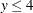### Presolver

PROC OPTMODEL includes a simple presolver that processes linear constraints to produce tighter bounds on variables. The presolver can reduce the number of variables and constraints that are presented to the solver. These changes can result in reduced solution times.

Linear constraints that involve only a single variable are converted into variable bounds. The presolver then eliminates redundant linear constraints for which variable bounds force the constraint to always be satisfied. Tightly bounded variables where upper and lower bounds are within the range specified by the VARFUZZ= option (see the section PROC OPTMODEL Statement) are automatically fixed to the average of the bounds. The presolver also eliminates variables that are fixed by the user or by the presolver.

The presolver can infer tighter variable bounds from linear constraints when all variables in the constraint or all but one variable have known bounds. For example, when given the following PROC OPTMODEL declarations, the presolver can determine the bound:

   proc optmodel;
var x >= 3;
var y;
con c: x + y <= 7;


The presolver makes multiple passes and rechecks linear constraints after bounds are tightened for the referenced variables. The number of passes is controlled by the PRESOLVER= option. After the passes are finished, the presolver attempts to fix the value of all variables that are not used in the updated objective and constraints. The current value of such a variable is used if the value lies between the variable’s upper and lower bounds. Otherwise, the value is adjusted to the nearer bound. The value of an integer variable is rounded before being checked against its bounds.

In some cases the solver might perform better without the presolve transformations, so almost all such transformations are unavailable when the option PRESOLVER=BASIC is specified. However, the presolver still eliminates variables that have values that have been fixed by the FIX statement. To disable the OPTMODEL presolver entirely, use PRESOLVER=NONE. The solver assigns values to any unused, unfixed variables when the option PRESOLVER=NONE is specified.